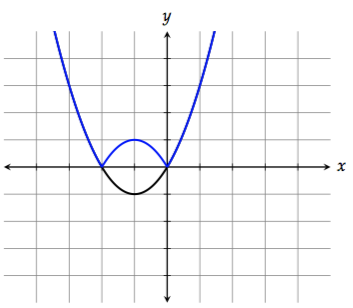# SAT ACT Math Rules Must Know

### SAT ACT math Rules – You must know before taking SAT/ACT Tests

SAT/ ACT Math Tutors in Dubai Abu Dhabi & Sharjah, We teach most important Rules along with Questions, sample of some common topics are shown below, also there are more specific topics by sub category.

1. Word Problems – 60% of the ACT/SAT math is word problems. More, less, fewer, higher, lower, double, half, etc.
2. Properties of Integers – 30% of Q’s used by the ACT/SAT are integers. Even, odd, positive, negative, consecutive, prime, factor, multiple, square, etc.
3. Rate- Time :  1/a + 1/b = (a + b)/ab. 1/2 + 1/3 = (2+3)/(2*3)
4. Sequences :  Arithmetic = add/subtract the Common Difference. Geometric = multiply/divide the Common Ratio, ‘n’th Term of sequence and Sum of ‘ n’ terms
5. Part+Part=Whole.
6. Rates & Proportions.
7. Increase, decrease, percent of a percent, Simple and Compound interest
8. Algebraic Operations & solving equations. Combine like terms – watch out for negative signs. Easy – don’t miss these.
9. Algebraic Inequalities – graph them quickly.
10. Absolute Value.
11. Exponents, roots, logarithms.
12. Linear Functions. ax+by=c & y=mx+b
13. Coordinate Geometry in the xy-plane.
14. Systems of Equations
15. Evaluate f(x).
16. Quadratics, parabolas, factor and FOIL.
17. Lines & Angles. l is parallel to m, l||m –> Same Slope
18. Triangles, right, isosceles, 3-4-5 and 5-12-13.
19. Quadrilaterals, squares, rectangles, trapezoids.
20. Circles & sectors. They love the angle of a piece of a pie chart.
21. Multiple Geometry Figures. Big Area – Small Area is usually the answer.
22. Two SOHCAHTOA questions, usually straightforward.
23. Average, Mean, Median, rarely mode.
24. Probability
25. Counting, Factorials, Permutations, & Combinations.

The graph of the function f is the graph of the function g compressed horizontally by a factor of 2 and reflected over the y-axis. Which of the following correctly defines the function f?

A) f(x) = g(-2x)
B) f(x) = -g(2x)
C) f(x) = 2 g(-x)
D) f(x) = -2 g(x)

we have to compress g horizontally by a factor of 2 and reflect it over the y-axis. Given what we’ve learned, this is pretty easy.

To compress the graph horizontally by a factor of 2, we replace x with 2x:  —>  g(2x)

To reflect the graph over the y-axis, we replace x with -x

The answer is A.

To understand the above Example in better way, please find the 7- Steps Method of same topic:

#### Step 1 ) f(x) = x^2 + 2x

whose graph looks like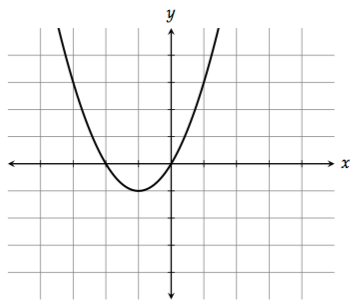#### Step 2 ) Shifting up and down

To shift the graph up, add a constant at the end of the function. For example, f(x) + 2 = x^2 + 2x + 2 would shift the graph up 2 units.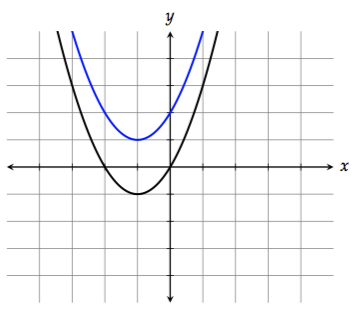To shift the graph down, subtract a constant at the end of the function. f(x) – 2 = x^2 + 2x – 2 would shift the graph down 2 units.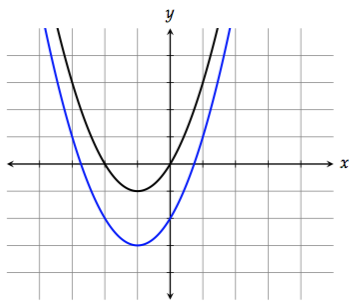#### Step 3 )  Shifting left and right

To shift the graph to the left by 1 unit, replace x with x + 1:

f(x + 1) = (x + 1)^2 + 2(x + 1)To shift the graph to the right by 1 unit, replace x with x – 1:

f(x – 1) = (x – 1)^2 + 2(x – 1)

The substitutions for left and right are counterintuitive to a lot of students because they’re the opposite of what you might expect.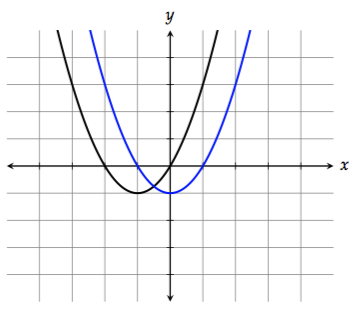#### Step 4 ) Reflecting across the x-axis

To reflect the graph across the x-axis (flip it upside down), multiply the function by -1:

-f(x) = -x^2 – 2x#### Step 5 )  Reflecting across the -axis

To reflect the graph across the y-axis, replace  with :

f(-x) = (-x)^2 + 2(-x) = x^2 – 2x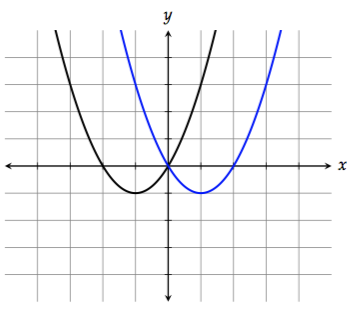#### Step 6)  Stretching/Compressing vertically

To stretch the graph vertically by a factor of 2, multiply  by 2:

2f(x) = 2(x^2 + 2x) = 2x^2 + 4x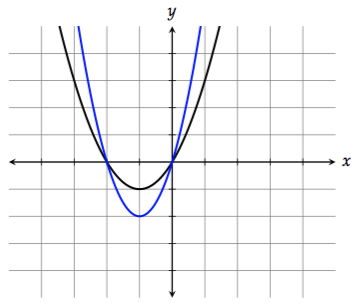You can think of this as the graph getting “taller.”

To compress the graph vertically by a factor of 2, multiply f(x) by  1\2:

1\2 * f(x) = 1/2 * (x^2 + 2x) = 1/2 * (x^2) + x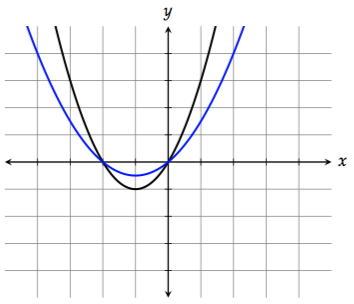Think of this as the graph getting “shorter.”

#### Step 7)  Stretching/Compressing horizontally

To stretch the graph horizontally by a factor of 2, replace x with ( 1\2 ) :

f ((1/2)x ) = ((1/2 )x)^2 + 2 *(1/2 )x = 1/4 * (x ^2) + x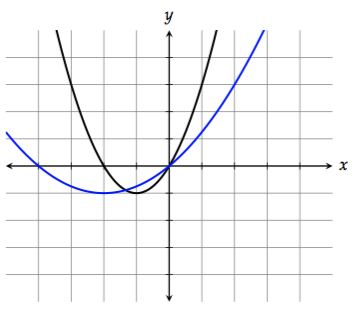Notice that the right side doesn’t get stretched as much. That’s because the points on the right side of the graph have smaller x-values (absolute value-wise). Doubling a small number doesn’t do as much as doubling a large number.

To compress the graph horizontally by a factor of 2, replace  with 2x:

f(2x) = (2x)^2 + 2(2x) = 4x^2 + 4x

By the way, these substitutions for horizontal stretch and compression are also counterintuitive to a lot of students. Be careful of horizontal transformations (shifting left and right, stretching/compressing). It’s often the opposite of what you might expect.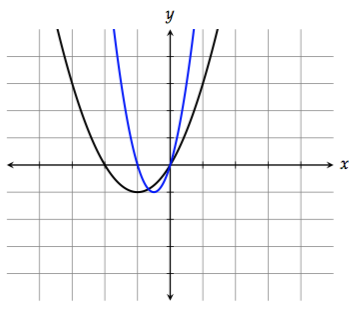Notice that the right side doesn’t get compressed as much. That’s because the points on the right side of the graph have smaller x-values (absolute value-wise). Halving a small number doesn’t do as much as halving a large number.

#### Absolute Value

To reflect all the points on the graph with negative y-values across the x-axis, take the absolute value of the function:

|f(x)| = |x^2 + 2 x